# Half Wave and Full Wave Precision Rectifier Circuit using Op-Amp

Published  April 29, 2020   3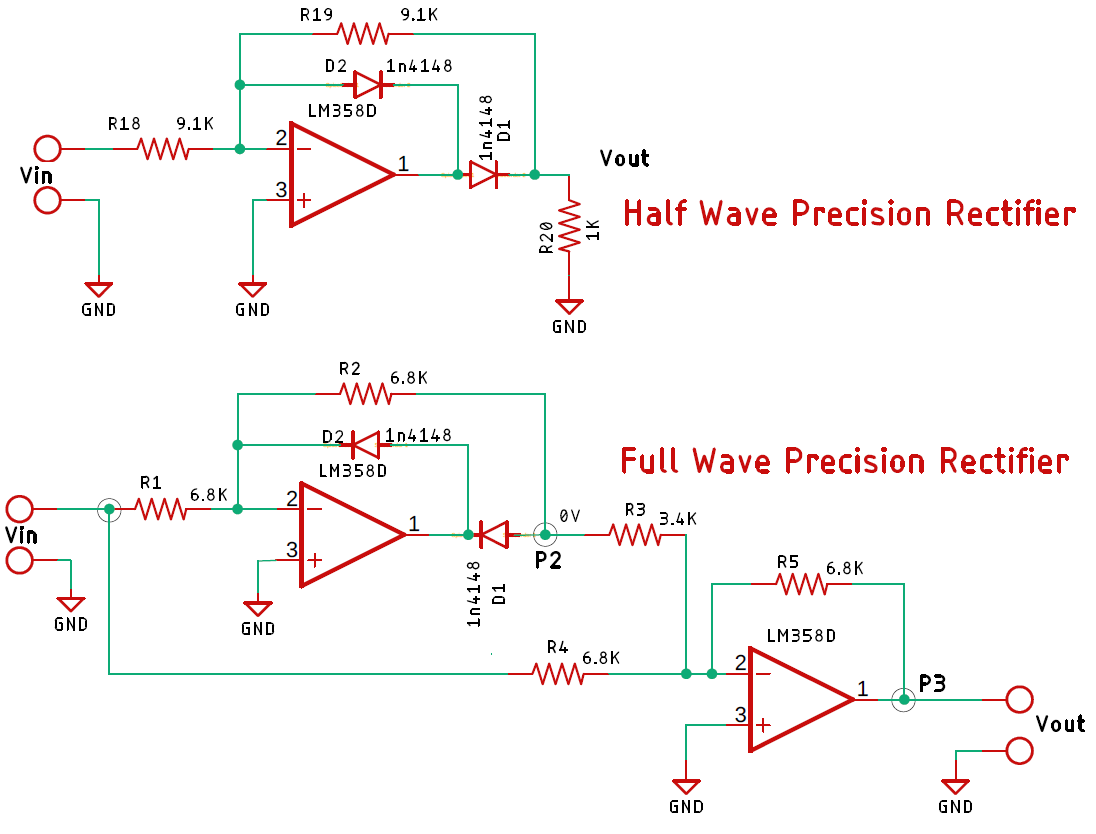A rectifier is a circuit that converts alternating current (AC) to Direct current (DC). An alternating current always changes its direction over time, but the direct current flows continuously in one direction. In a typical rectifier circuit, we use diodes to rectify AC to DC. But this rectification method can only be used if the input voltage to the circuit is greater than the forward voltage of the diode which is typically 0.7V. We previously explained diode-based half-wave rectifier and full-wave rectifier circuit.

To overcome this issue, the Precision Rectifier Circuit was introduced. The precision rectifier is another rectifier that converts AC to DC, but in a precision rectifier we use an op-amp to compensate for the voltage drop across the diode, that is why we are not losing the 0.6V or 0.7V voltage drop across the diode, also the circuit can be constructed to have some gain at the output of the amplifier as well.

So, in this tutorial, I am going to show you how you can build, test, apply, and debug a precision rectifier circuit using op-amp. Alongside that, I will be discussing some pros and cons of this circuit as well. So, without further ado, let's get started.

### What is a Precision Rectifier Circuit?

Before we know about the Precision Rectifier Circuit, let's clarify the basics of the rectifier circuit.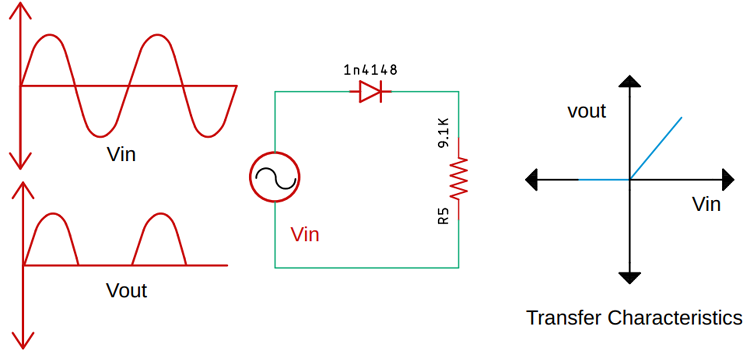The above figure shows the characteristics of an ideal rectifier circuit with its transfer characteristics. This implies when the input signal is negative, the output will be zero volts and when the input signal is positive the output will follow the input signal.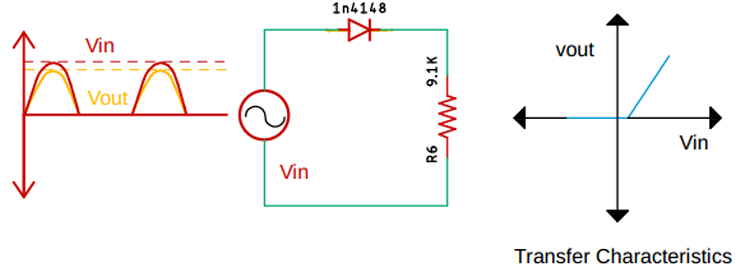The above figure shows a practical rectifier circuit with its transfer characteristics. In a practical rectifier circuit, the output waveform will be 0.7 volts less than the applied input voltage, and the transfer characteristic will look like the figure shown in the diagram. At this point, the diode will only conduct if the applied input signal is slightly greater than the forward voltage of the diode.

Now the basics out of the way, let's turn our focus back to the precision rectifier circuit.

### Working of Precision Rectifier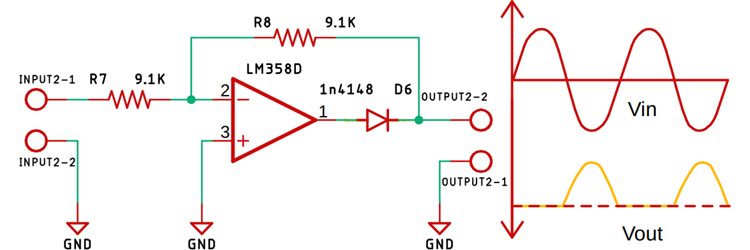The above circuit shows a basic, half-wave precision rectifier circuit with an LM358 Op-Amp and a 1n4148 diode. To learn how an op-amp works, you can follow this op-amp circuit.

The above circuit also shows you the input and output waveform of the precision rectifier circuit, which is exactly equal to the input. That's because we are taking the feedback from the output of the diode and the op-amp compensates for any voltage drop across the diode. So, the diode behaves like an ideal diode.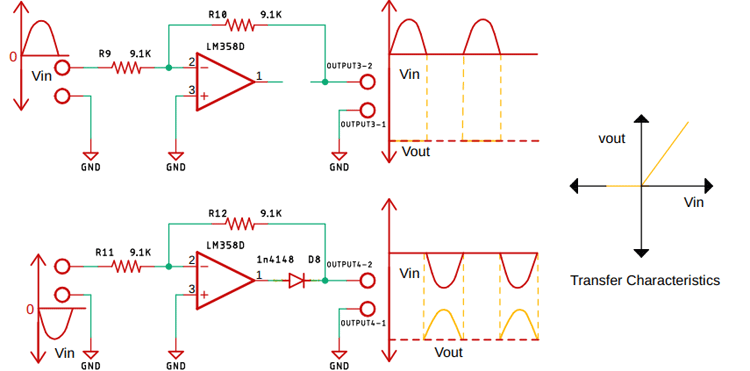Now in the above image, you can clearly see what happens when a positive and a negative half cycle of the input signal is applied in the input terminal of the Op-Amp. The circuit also shows the transfer characteristics of the circuit.

But in a practical circuit, you will not get the output as shown in the above figure, let me tell you why?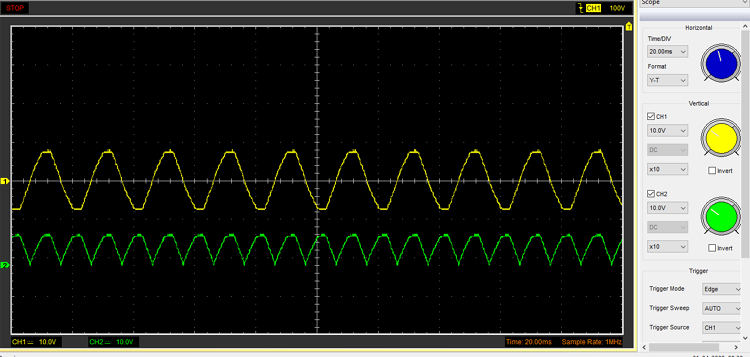In my oscilloscope, the yellow signal in the input, and the green signal is the output. Instead of getting a half-wave rectification, we are getting a sort of full-wave rectification.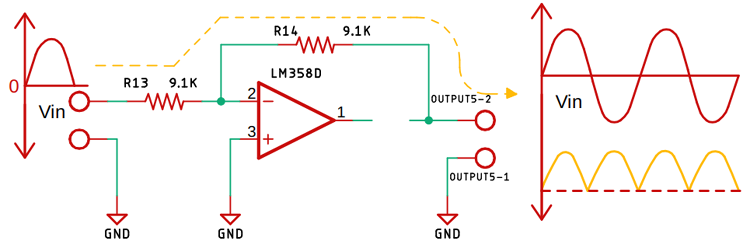The above image shows you when the diode is off, the negative half cycle is of the signal flows through the resistor on to the output, and that is why we are getting the full-wave rectification like the output, but this is not the actual case.

Let's see what happens when we connect a 1K load.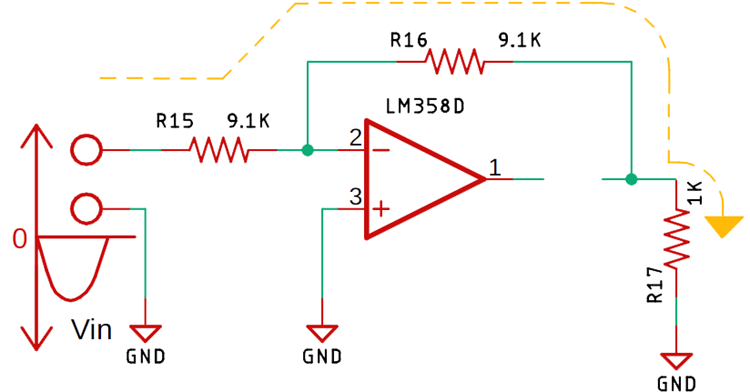The circuit looks like the above image.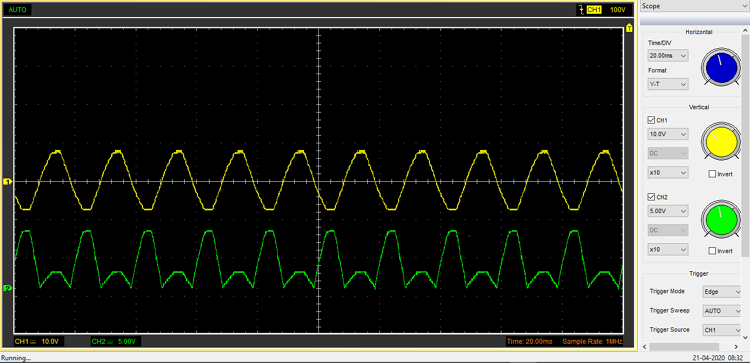The output looks like the above image.

The output looks like this because we have practically formed a voltage divider circuit with two 9.1K and a 1K resistor, that is why the input positive half of the signal just got attenuated.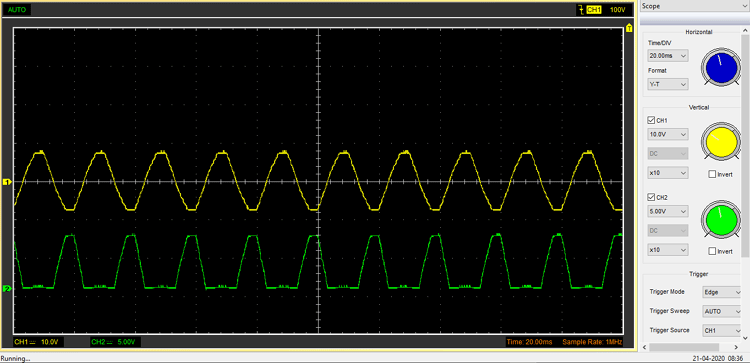Again, this above image shows you what happens when I change the load resistor value to 220R from 1K.

This is not the least bit of a problem that this circuit has.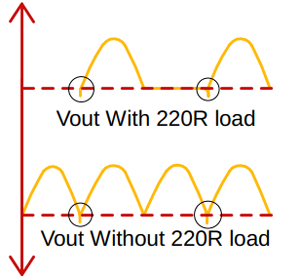The above image shows you an undershoot condition where the output of the circuit goes below zero volts and rises after a certain spike.

The above image shows you an undershoot condition for both of those above-mentioned circuits, with load and without load. That is because, whenever the input signal goes below zero, the op-amp goes into the negative saturation region and the result is the shown image.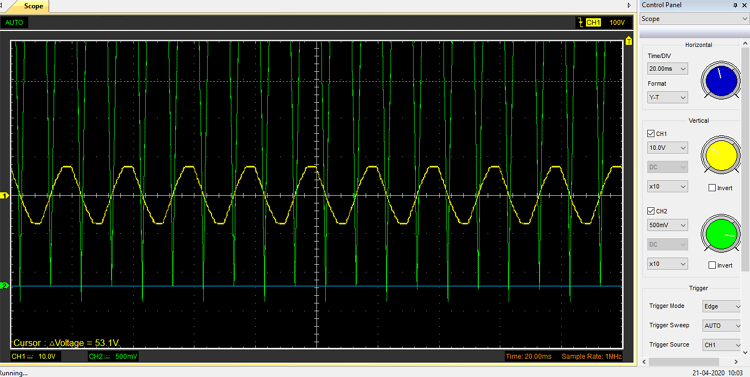Another reason we can say that, whenever the input voltage swings from positive to negative, it will take some time before the op-amps feedback comes into play and stabilizes the output, and this is why we are getting the spikes below zero volts on the output.

This is happening because I am using a jelly bean LM358 op-amp with a low slew rate. You can get away with this problem, just by putting an op-amp with a higher slew rate. But keep in mind that this will also happen in the higher frequency range of the circuit.

### The Modified Precision Rectifier Circuit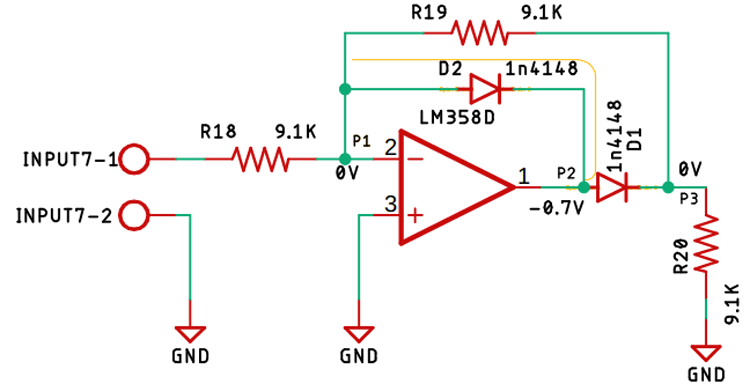The above figure shows a modified precision rectifier circuit through which we can reduce all the above-mentioned flaws and drawbacks. Let's study the circuit and figure out how it works.

Now in the above circuit, you can see that the diode D2 will conduct if the positive half of the sinusoidal signal is applied as an input. Now the above-shown path (with the yellow line) is completed and the Op-amp is acting as an inverting amplifier, if we look at point P1, the voltage is 0V as a virtual ground is formed at that point, so current cannot flow through the resistor R19, and in the output point P2, the voltage is negative 0.7V as the op-amp is compensating for the diode drop, so there is no way that current can go to point P3. So, that is how we have achieved a 0V output whenever a positive half cycle of the signal is applied to the input of the Op-amp.

Now let us assume that we have applied the negative half of the sinusoidal AC signal to the input of the op-amp. That means the applied input signal is less than 0V.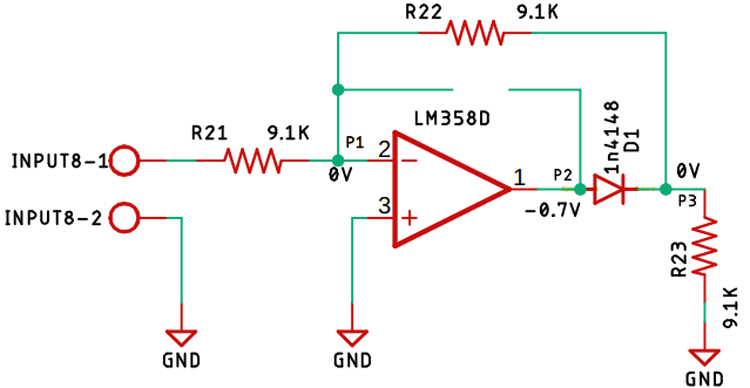At this point, the Diode D2 is in the reverse-biased condition that means it's an open circuit. The image above exactly tells you that.

As the Diode D2 is in the reverse-biased condition, the current will flow through the resistor R22 forming a virtual ground at point P1. Now when the negative half of the input signal is applied, we will get a positive signal in the output as its an inverting amplifier. And the diode will conduct and we will get the compensated output at point P3.

Now the output voltage will be -Vin/R2 = Vout/ R1

So the output voltage becomes  Vout = -R2/R1* Vin

Now let us observe the output of the circuit in the oscilloscope.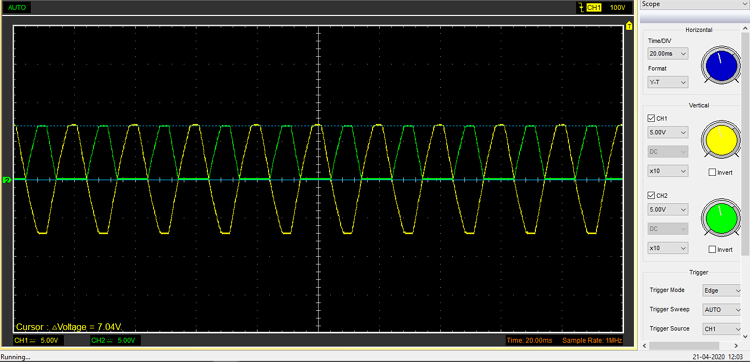The practical output of the circuit without any load attached is shown in the above image.

Now when it comes to the analysis of the circuit, a half-wave rectifier circuit is good enough, but when it comes to a practical circuit, the half-wave rectifier just does not make practical sense.

Because of that reason, a full-wave rectifier circuit was introduced, to achieve a full-wave precision rectifier, I just need to make a summing amplifier, and that's basically it.

### Precision Full Wave Rectifier using Op-Amp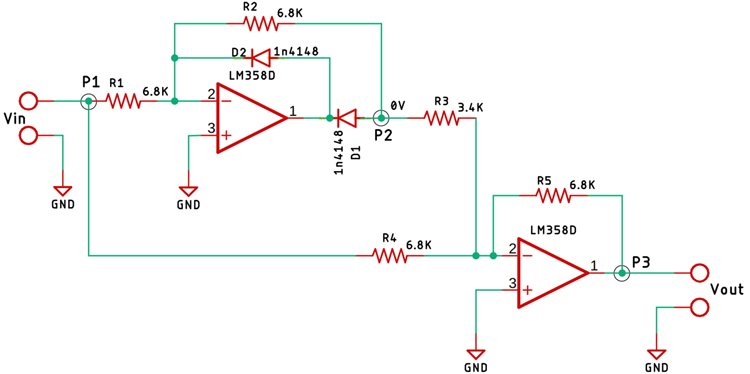To make a full-wave precision rectifier circuit, I have just added a summing amplifier to the output of the previously mentioned half-wave rectifier circuit. From the point, P1 to point P2 is the basic precision rectifier circuit and the diode is so configured that we get a negative voltage at the output.

From the point, P2 to point P3 is the summing amplifier, the output from the precision rectifier is fed to the summing amplifier through the resistor R3. The value of the resistor R3 is half of R5 or you can say it’s R5/2 that is how we are setting a 2X gain out of the op-amp.

The input from the point P1 is also fed to the summing amplifier with the help of the resistor R4, the resistors R4 and R5 are responsible for setting the gain of the op-amp to 1X.

Since the output from the Point P2 is fed directly to the summing amplifier with the gain of 2X, that means the output voltage will be 2-times the input voltage. Let's assume the input voltage is 2V peak, so we will get a 4V peak at the output. At the same time, we are directly feeding the input to the summing amplifier with a gain of 1X.

Now when the summing operation happens we get a summed up voltage at the output which is (-4V) + (+2V) = -2V and as the op-amp at the output. As the op-amp is configured as an inverting amplifier, we will get +2V at the output which is the point P3.

The same thing happens when the negative peak of the input signal is applied.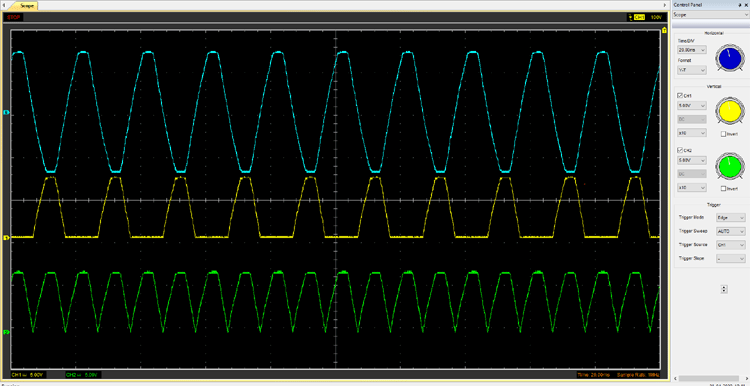The above image shows the final output of the circuit, the waveform in blue is the Input and the waveform in Yellow is the output from the half-wave rectifier circuit, and the waveform in green is the output of the full-wave rectifier circuit.

### Components Required

• LM358 op-amp IC - 2
• 6.8K, 1% Resistor - 8
• 1K Resistor - 2
• 1N4148 Diode - 4
• Jumper Wires - 10
• Power Supply (± 10V) - 1

### Schematic Diagram

Circuit diagram for half-wave and full-wave precision rectifier using op-amp is given below: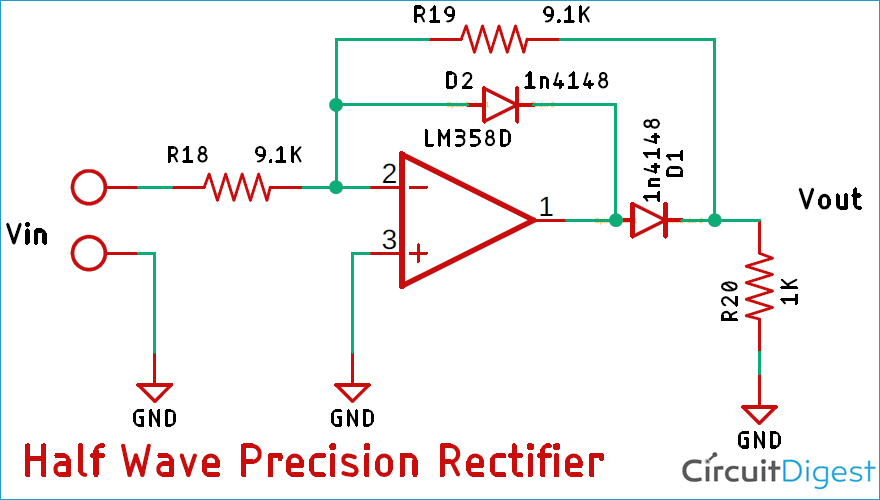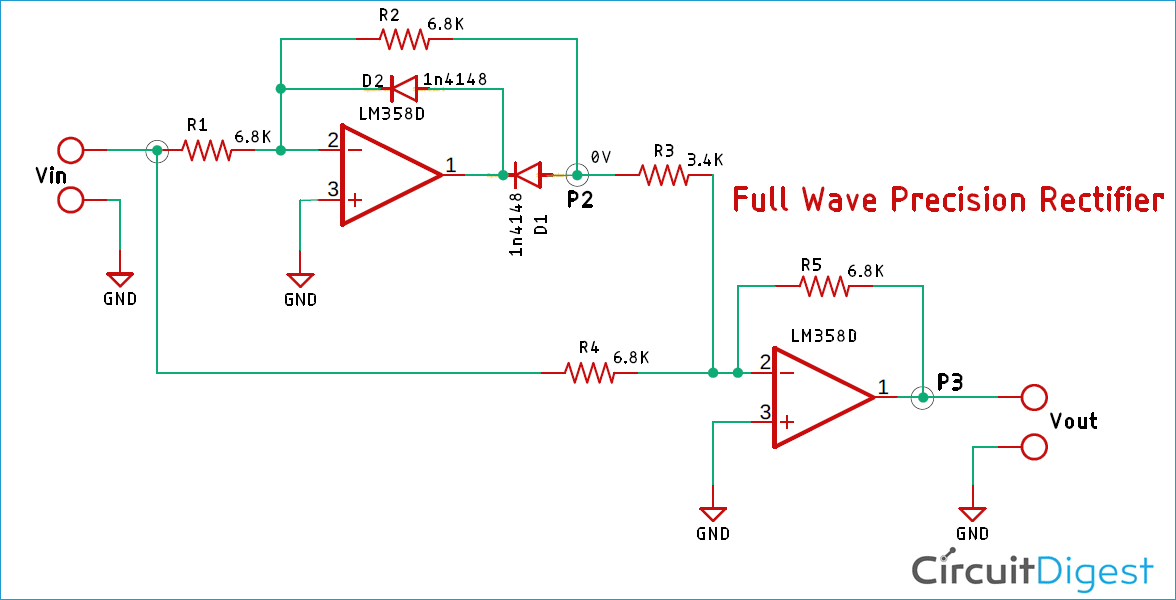For this demonstration, the circuit is constructed in a solderless breadboard, with the help of the schematic; To reduce parasitic inductance and capacitance, I have connected the components as close as possible.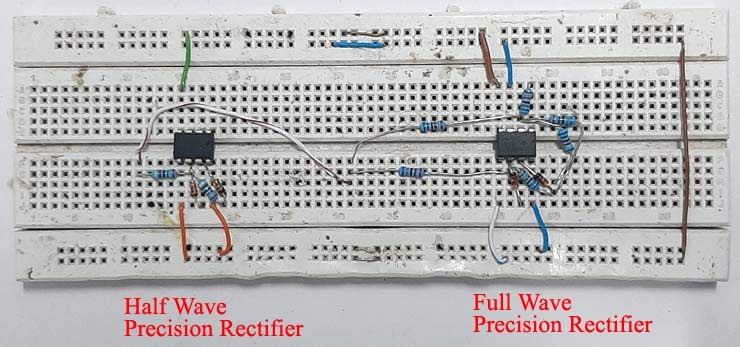### Further Enhancement

The circuit can be further modified in order to improve its performance like we can add an additional filter in order to reject high-frequency noises.

This circuit is made just for demonstration purposes only. If you are thinking about using this circuit in a practical application, you have to use a chopper type op-amp and high precision 0.1 ohms resistor to achieve absolute stability.

I hope you liked this article and learned something new out of it. If you have any doubt, you can ask in the comments below or can use our forums for detailed discussion.

Video

### Well explained, but errors in text

Well explained Debashis, but look at the part where you mention

"Now the output voltage will be -Vin/R2 = Vout/ R1

So the output voltage becomes  Vout = -R2/R1* Vin".

There are no such resistors! For someone familiar with how op-amps work, this is not difficult to figure out, but your description needs to be consistent with component names used.